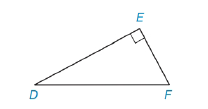Chapter 5.4, Problem 8EElementary Geometry For College St...

7th Edition
Alexander + 2 others
ISBN: 9781337614085

Solutions

Chapter
SectionElementary Geometry For College St...

7th Edition
Alexander + 2 others
ISBN: 9781337614085
Textbook Problem

Find the length of D E ¯ if:a) D F = 13   and E F = 5 .b) D E = 12 and E F = 6 3 .Exercises 7-10

To determine

(a)

To Find:

The length of DE¯.

Explanation

Given DF=13 and EF= 5,

And figure,

From the figure,

ΔDEF is a right triangle with right angle E

From the Pythagorean Theorem,

The square of the length of hypotenuse of a right triangle is equal to the sum of the square of the lengths of the other two sides.

From the figure, DF¯ is the hypotenuse,

DF2=EF2+D

To determine

(b)

To Find:

The length of DE¯.

Still sussing out bartleby?

Check out a sample textbook solution.

See a sample solution

The Solution to Your Study Problems

Bartleby provides explanations to thousands of textbook problems written by our experts, many with advanced degrees!

Get Started